# Common Core: High School - Number and Quantity : Rational Exponents: CCSS.Math.Content.HSN-RN.A.1

## Example Questions

← Previous 1

### Example Question #1 : Rational Exponents: Ccss.Math.Content.Hsn Rn.A.1

Evaluate: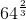Explanation:

To evaluate this, let's rewrite the problem.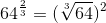Now lets break down the cube root.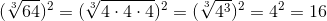### Example Question #2 : Rational Exponents: Ccss.Math.Content.Hsn Rn.A.1

Evaluate: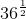Explanation:

To evaluate this, let's rewrite the problem.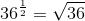Now lets break down the square root.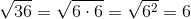### Example Question #1 : Rational Exponents: Ccss.Math.Content.Hsn Rn.A.1

Evaluate: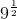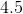Explanation:

To evaluate this, let's rewrite the problem.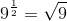Now lets break down the square root.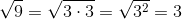### Example Question #1 : High School: Number And Quantity

Evaluate: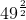Explanation:

To solve this, let's reduce the exponent, and then solve.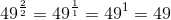### Example Question #5 : Rational Exponents: Ccss.Math.Content.Hsn Rn.A.1

Evaluate: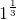Explanation:

One to any power is just, so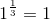### Example Question #6 : Rational Exponents: Ccss.Math.Content.Hsn Rn.A.1

Evaluate: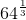Explanation:

To evaluate this, let's rewrite the problem.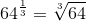Now lets break down the cube root.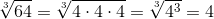### Example Question #2 : High School: Number And Quantity

Evaluate: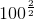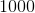Explanation:

To solve this, let's reduce the exponent, and then solve.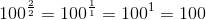### Example Question #8 : Rational Exponents: Ccss.Math.Content.Hsn Rn.A.1

Evaluate: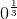Explanation:

Zero to any power is just, expect when the power is. So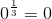### Example Question #1 : High School: Number And Quantity

Evaluate: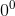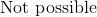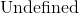Explanation:

Zero raised to the zero is equal to one, otherwise it is equal to zero.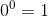### Example Question #10 : Rational Exponents: Ccss.Math.Content.Hsn Rn.A.1

Evaluate: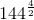Explanation:

The first part to solving this problem is reducing the exponent, and then solving.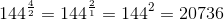← Previous 1

### All Common Core: High School - Number and Quantity Resources